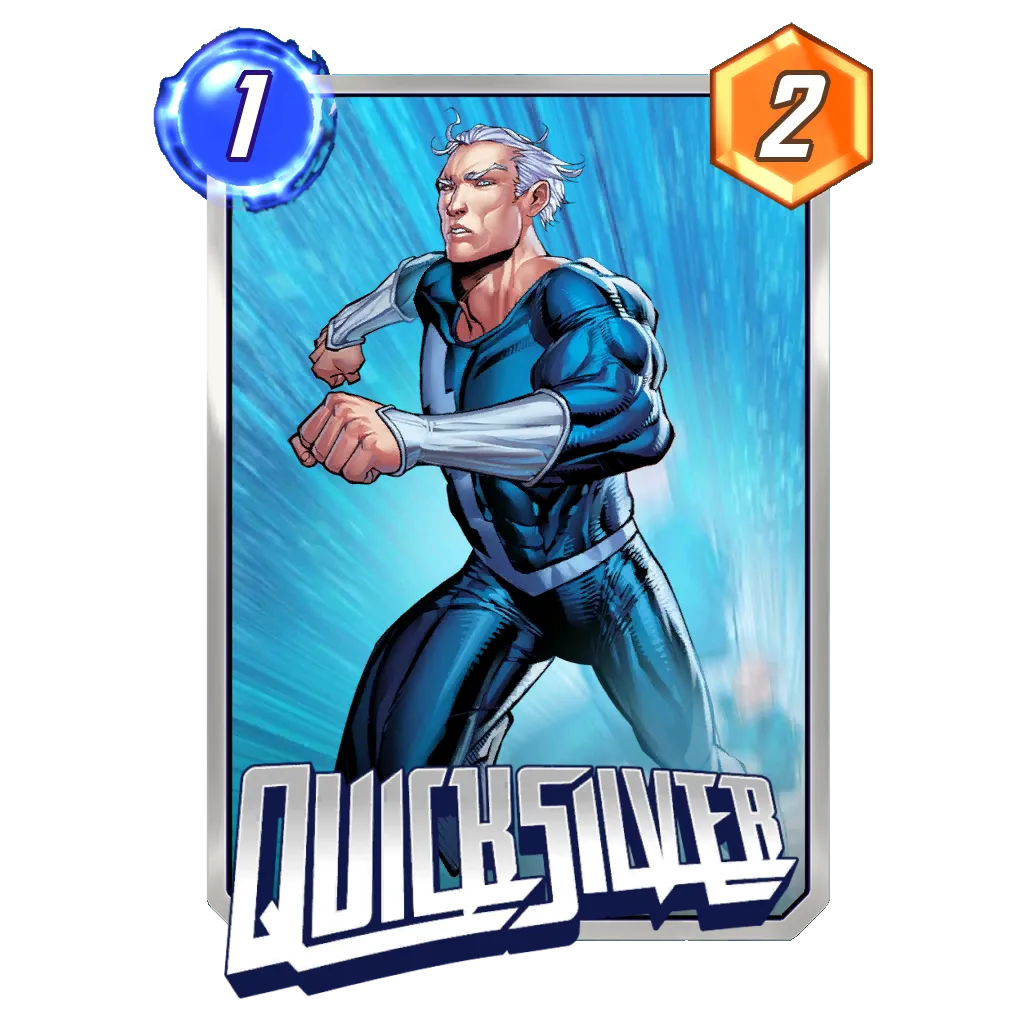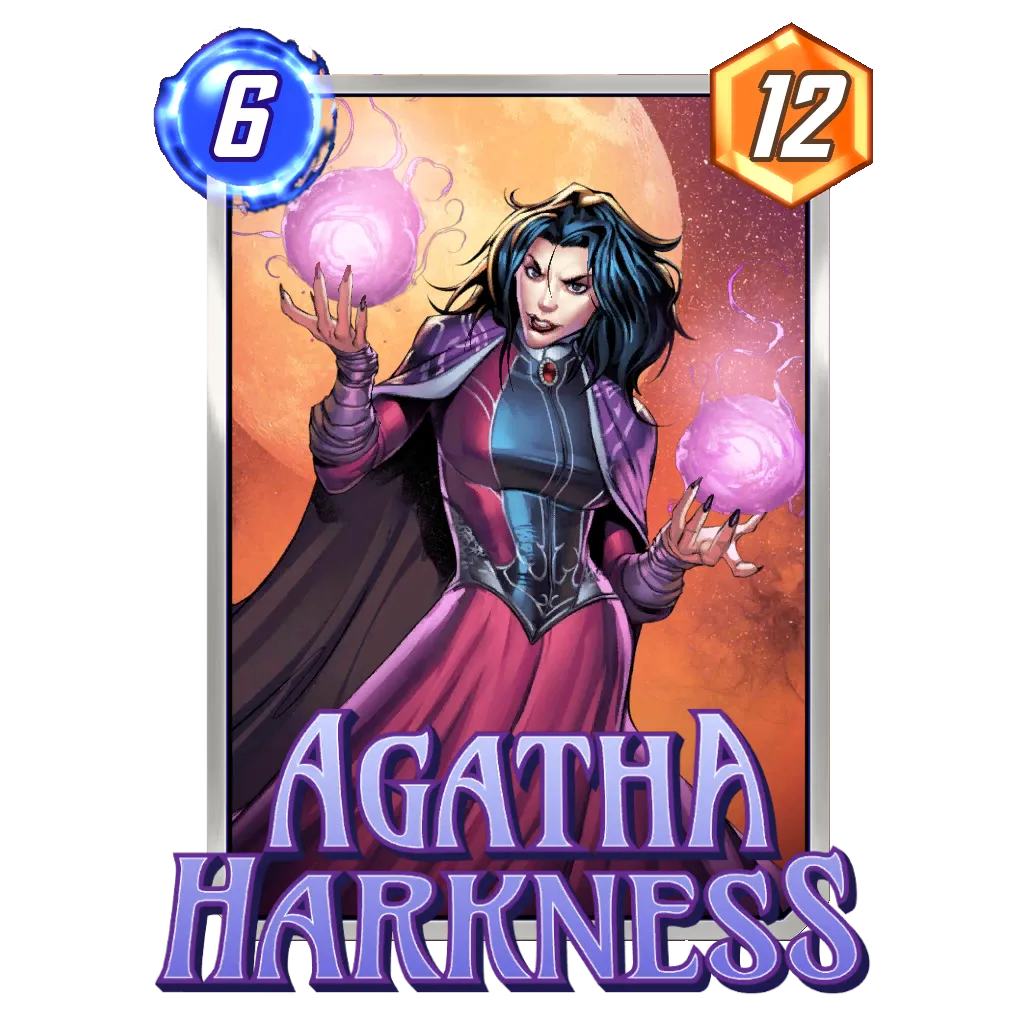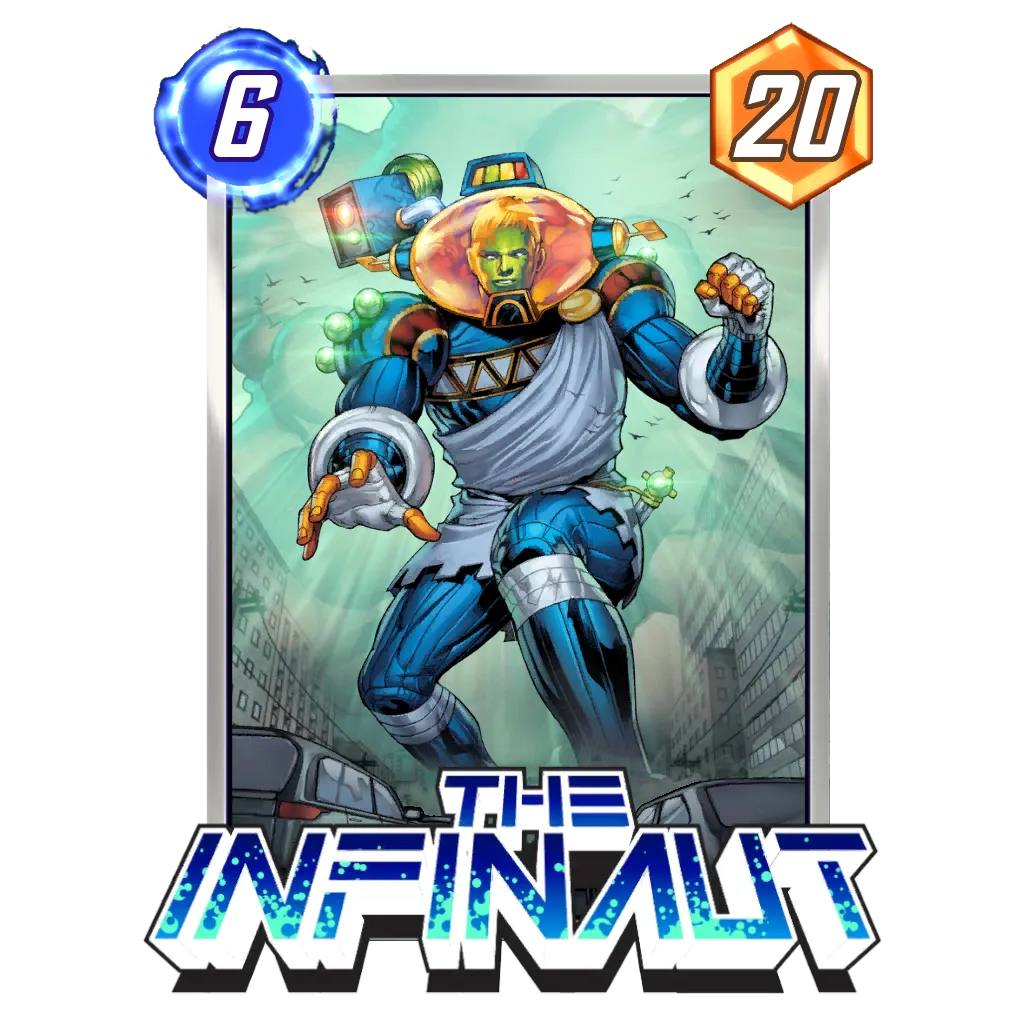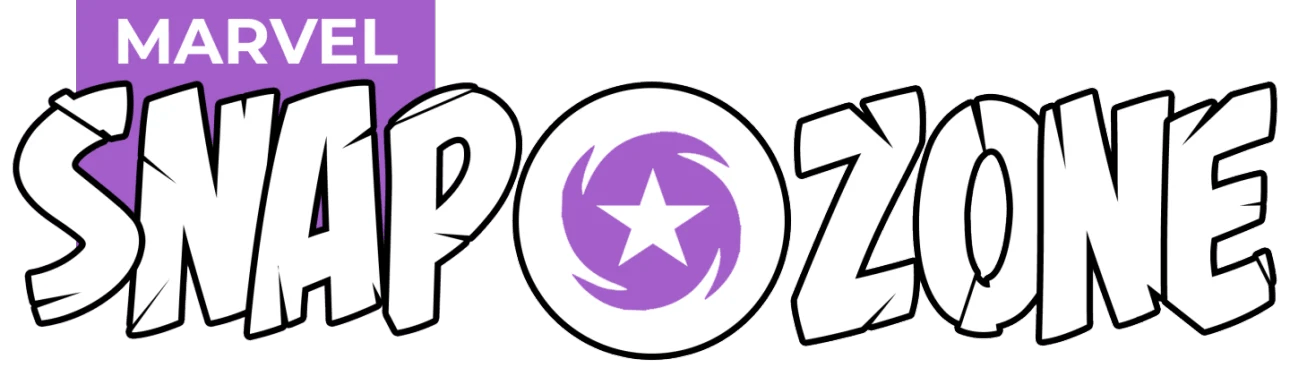# How to Calculate Card Drawing Probabilities in Marvel SnapNot everyone is as lucky as Domino: Know your probability to draw a card in Marvel Snap.

I recently saw a video about the some probabilities in Marvel Snap and also saw that some people enjoyed it, so I thought some people could need help learning some of the basic probabilities in the game and write a guide on how to calculate the card draw Probability in Marvel Snap.

This guide will not only give you the probabilities for drawing specific cards, but will also teach you how one can calculate them, but will not include basics on how to play.

Everything here is without having Rocks added to your deck (from locations or Korg) etc. but if you understand this guide, you will be able to calculate these probabilities yourself. 😉

The first part of this guide will be the theory including some tables with the calculated probabilities.

Part two will include some example of how one could use this for building a specific deck.

The guide only focuses on drawing single cards or specific mana drops on curve and not combos (this might be shown in a future guide). It is written for Marvel Snap, but the principles behind it can be used for other card games as well.

Note: I do NOT round probabilities here, I normally leave the periodic part at the end instead.

## Basics and assumptions to calculate the probabilities

Before going more into detail, I want to write down the basics as well as the assumptions.

This is important in case in the future parts of the game changes (and thus understanding why this guide made sense in the past), but also to understand the basic principles of probabilities which will help you to apply the learned to other card games.

• You are running a 12 card deck, with every card once in there.
• You start with 3 cards in your hand, and you draw every turn exactly one additional card.
• The game lasts normally 6 turns.
• Each card in your deck is unique (so no duplicates)
• Each turn you have mana equal to the turn number (1 in the first turn, 2 in the second and so on).
• You normally want to fully utilize all mana you have in a turn for maximum gain.

From this we can get the basic probabilities of drawing a specific card:

#### TABLE 1: Basic Probability to draw a specific card in Marvel Snap

• On turn 0 (before you draw on first turn) you have 3 out of12 cards drawn. This gives the probability of 3/12 = 1/4 = 25% to have drawn a specific card. You can just calculate this probability by dividing the number of cards drawn by the number of total cards.
• on turn 1 you have 4/12 cards drawn this gives the probability of 4/12 = 1/3 = 33%
• on turn 2 you have 5/12 cards drawn -> 41.66% probability for a card
• on turn 3 you have 6/12 cards drawn -> 1/2 = 50% probability
• on turn 4 7/12 -> 58.33%
• on turn 5 8/12 -> 66.66% = 2/3
• on turn 6 9/12 -> 75.00% = 3/4
• on turn 7 10/12 -> 83.33% = 5/6 (or on turn 6 if drawing an additional card)
• on turn 8 11/12 -> 91.66% (Or on turn 6 if drawing 2 additional cards)
• on turn 9 12/12 -> 100% You have drawn all caards in your deck!

I mention turn 7, 8 and 9 not only because it could happen with new locations in the future, but also since drawing additional cards (by location effects (or by future cards) will have the same effect as being in a future turn.

So if you draw 1 additional card and are in turn 3 you have the same probability to draw a specific card as the base probability (if you would not have drawn an additional card) you have in turn 4.

If you want to learn more about combinatorics Wikipedia can help.

## The Probability to have one of several X drops on turn XThe probability to draw this card is easy to calculate.

What I mean here is that have 3 one-drops (cards costing 1 mana) in your deck and want to know the chances you have at least 1 one-drop on in your hand on turn 1.

The basic math operation covering that is “choose” it gives you the answer to the question:

How many different combinations are there to draw X cards out of Y cards.

• Hint: you can type into most search engines (like google or duckduckgo etc.) 12 choose 4 and it gives you the result. So its easy to calculate yourselves!
• So for example the number of different starting hands on turn 1 (including first card) is: 12 Choose 4 = (1211109)/(4321)= 495
• So lets say you have 3 one drop cards. Now lets count the number of different starting hands which do not contain a one drop This is 12 minus 3 (3 cards you dont want to draw) choose 4. So 9 choose 4= 126
• This means 465-126=369 different starting hands out of the 465 have a 1 drop. Which is 369/495=74.545% chance of drawing one of the 1 drops on turn 1

So lets do the same for other numbers and turns.

#### TABLE 2: Probability to draw X mana card on curve in Marvel Snap

• One Drop on turn 1:
• 1 One drop: 4/12= 33.3% (same as single card)
• 2 One drops: (495-((12-2) choose 4))/495 =57.57%
• 3 One drops: 74.545% (see above)
• 4 One drops: (495-((12-4) choose 4))/495 = 85.85%
• 5 One drops: (495-((12-5) choose 4))/495 = 92.92%
• 6 One drops: (495-((12-6) choose 4))/495 = 96.96%
• 7 One drops: (495-((12-7) choose 4))/495 = 98.98%
• 8 One drops: (495-((12-8) choose 4))/495 = 99.79%
• 9 One drops: 100% (you draw 4 cards from 12 and only 3 are no 1 drops)
• Two Drops on turn 2:
• Number of hands turn 2= 12 choose 5 = 792
• 1 Two drop: 5/12= 41.66%
• 2 Two drops: (792 -((12-2) choose 5))/792 = 68.18%
• 3 Two drops: (792 -((12-3) choose 5))/792 = 84.09%
• 4 Two drops: (792 -((12-4) choose 5))/792 = 92.92%
• 5 Two drops: (792 -((12-5) choose 5))/792 = 97.348%
• 8 Two Drops: 100%
• Three Drops on turn 3:
• Number of Hands turn 3= 12 choose 6 = 924
• 1 Three drop: 6/12= 50%
• 2 Three drops: (924-((12-2) choose 6))/924= 77.27%
• 3 Three drops: (924-((12-3) choose 6))/924= 90.90%
• 4 Three drops: (924-((12-4) choose 6))/924= 96.96%
• 7 Three drops: 100%
• Four Drops on turn 4:
• Number of Hands turn 4= 12 choose 7 = 792 (because thats the same as 12 choose 5 (the 5 cards you have not drawn)
• 1 four drop: 7/12= 58.3%
• 2 four drops: (792-((12-2) choose 7))/792= 84.84%
• 3 four drops: (792-((12-2) choose 7))/792= 95.45%
• 6 four drops: 100%
• Five Drops on turn 5:
• Number of Hands turn 5= 12 choose 8 = 495
• 1 five drop: 8/12= 66.66%
• 2 five drops: (495-((12-2) choose 8))/495= 90%
• 3 five drops: (495-((12-3) choose 8))/495= 98.18%
• 5 Fife drops: 100%
• Six Drops on turn 6:
• Number of Hands turn 6= 12 choose 9 = 220
• 1 six drop: 9/12= 75%
• 2 six drops: (220-((12-2) choose 9))/220= 95.45%
• 4 six drops: 100%

This may help you a bit to better know what kind of distribution could help to make your deck more consistent. It shows nicely why 1 6 drop is most often enough, since this gives you a pretty high chance of drawing it. And adding a second does increase the chance only by 20% where for other spots the probabilities can increase more. (Also on turn 6 you also have a good chance of having drawn a second 5 drop +1 drop or 4 drop +2 drop etc. to fill your curve).

You can also use resources like this one or this one to calculate certain probabilities, if you do not want to calculate it yourselves.

## Cards which are drawn on a fixed turn

There are several effects in Marvel Snap, which let you draw a certain card at a certain turn.

At the moment there are 2 cards which are drawn to the opening hand (turn 0), one card is drawn on turn 2 and one is drawn on turn 6.

Having these cards does change your probabilities to draw cards of course. To take a closer look at this, lets first take a look at the following hypothetical card:

### Cards which are drawn later/never drawnThis Marvel Snap card improves the probability to draw other cards early!

At the moment we have America Chavez, which for most turns (except turn 6) pretty much just comes down to having a card which can’t be drawn. Nevertheless for completeness lets consider the following theoretical card:

The end:
This card is drawn on turn 10. If you draw it you lose the game.

So this card will never be drawn, except in special circumstances.

So lets look how this changes the probability to draw a specific card until the specific turn:

#### TABLE 3: One card less to draw.

• Turn 0: 3/11 = 27.27%
• Turn 1: 4/11 = 36.36%
• Turn 2: 5/11= 45.45%
• Turn 3: 6/11 = 54.54%
• Turn 4: 7/11 = 63.63%
• Turn 5: 8/11 = 72.72%
• Turn 6: 9/11 = 81.81% (This would be different for America Chavez, since you always draw her on this turn)
• Turn 7: 10/11 = 90.90%
• Turn 8: 11/11 = 100%

As you can see this improves the probabilities a bit, especially for drawing a card until turn 5, the probability is increased by 8/11-8/12=6.06%.

This may not sound like a lot, but it means its more than 6 out of 100 games.

#### How would it look like with 2 cards like America Chavez?

This is mostly not relevant (except for turn 0/1), but lets look how these probabilities change, when you would have two cards “The end” (the example above, which is never drawn) in your deck:

#### Table 4: Two cards less to draw (theoretical)

• Turn 0: 3/10 = 30%
• Turn 1: 4/10 = 40%
• Turn 2: 5/10= 50%
• Turn 3: 6/10 = 60%
• Turn 4: 7/10 = 70%
• Turn 5: 8/10 = 80%
• Turn 6: 9/10 = 90%
• Turn 7: 10/10 = 100%

So when would this be relevant?

Well at the moment, the only way this would ever be relevant is for turn 1, if you play America Chavez and Domino.

Then the chance of drawing a specific card on turn 1 increases from 33% to 40%, but maybe in the future other cards make this more relevant.

### Card is already drawn, Quicksilver, Domino and Agatha Harkness:Always drawing Agatha means you draw other cards less often!

Now lets take a look at Quicksilver, Domino (and America Chavez).

So how does it change the probabilities to draw cards the turn when they are drawn and after?

Well its quite simple. You just draw 1 card less, but you can use the same probabilities as in the first table above (“Important Table”), just go up one.

So with Quicksilver the chances of drawing the card on turn 1 are the same as with “the end” drawing it on turn 0 and so on.

If you would have both Quicksilver AND Domino, you can use the second table, but go back 2 turns.

So the chances of drawing a card on turn 4 with Quicksilver and Domino are the same as drawing it with 2 “The Ends” on turn 2. (The hypothetical 2nd table).

## Example on how to use probabilities: The Infinaut deckYou want to draw The Infinaut before the last turn!

This is a quite good example, because I will show the following:

If you want to play a Deck with The Infinaut, you should also play America Chavez

• This sounds a bit counter intuitive when you hear it, because The Infinaut is a 6 drop and Americ Chavez as well, and it makes sure that you cannot draw your wanted 6 drop on turn 6.
• So lets look at the probabilities to draw The Infinaut with America Chavez and without. Without the probability to get The Infinaut by turn 6 is 75%. With it is only 72.72% so you actually lose 2.27% !
• This is true, however, lets now look at the probability to draw The Infinaut by turn 5: Without America Chavez it is: 66.66% and with America Chavez it is 72.72% so in the 2nd case it is 6.06% higher
• This means that Without America Chavez in 75%-66-66%=8.3% of the cases you draw The Infinaut in your last turn. The problem here is that in 25% of all games you do not draw The Infinaut at all.
• This means if you do not have The Infinaut in your hand on turn 5 only in 1/4 cases you actually draw it in the last turn. So if you gable about drawing it, you lose the gamble in 3/4 games.
• It may be still better to gamble for this, since you will lose the games without The Infinaut anyway, but this does not have to be the case.
• And if you do not want to take this gamble, and play things on turn 5, you will draw a card on turn 6, which you cannot play at all! Which might suck, if you don’t have enough cards to spend the mana!
• This means with America Chavez in your deck, you will have The Infinaut more often turn 5 (such that you can actually plan with it) and in the 27% of the games you do not have it, you know the next turn you draw 10 power for 6 mana, so you can plan with this.
• This means you have a 6.06% higher chance to actually have a “good game” and your “bad games” (where you don’t draw the key card), got better since you know exactly what you draw on turn 6 (and its 10 power which is not too bad).
• Additional if you play other important cards (like sun spot), you also have a 5.3% higher chance to draw them till turn 4.

### About playing Domino in this Deck:

This would increase the chance of drawing a specific card on turn 1 a bit. (Sunspot as one example) and not decrease the probability to draw The Infinaut too much (2.7%), but I do not think this would be worth it, since The Infinaut is really important, and you are fine with drawing Sunspot later (turn 2, 3, 4).

You might even prefer to draw it later, because then its less in danger to be destroyed (Elektra), when you play it later.

### General Mana curve for the Deck:

I would in this deck play a lower than normal mana curve, since you do no want 5 drops at all, and in case you cant use all mana each turn, you have also sunspot which might even use that mana.

Additional there are 2 cases: First case is when you draw The Infinaut, then you want to play as many (good) cards as you can on the first 4 turns, since you can’t play cards on turn 5.

The 2nd case is, when you have not drawn The Infinaut, then you know you can dump the whole hand on turn 5, since you will be drawing a 6 drop the next turn and can’t use additional mana.

Additional with (often) not being able to play anything on turn 5, you might want to make sure to get the best from turn 1 at least.

So I would play most likely 4 1 drops (including Sunspot), which gives a 89.39% chance of having a 1 drop on turn 1.

#### TABLE 5: probability to draw X mana card on curve in “The Infinaut Deck” in Marvel Snap

One drop on turn 1:

• 11 choose 4 = 33
• 1 One drop: 4/11= 36.36%
• 2 One drops: (330-((11-2) choose 4))/330 = 61.81%
• 3 One drops: (330-((11-3) choose 4))/330 = 78.78%
• 4 One drops: (330-((11-4) choose 4))/330= 89.39% (Quicksilver would be a 100% chance, but we don’t want him, since he decreases the probability for sun spot and The Infinaut).

For an optimal curve playing 3 or 4 one drops would be optimal in this deck.

Two Drop on turn 2:

• 11 choose 5 = 462
• 1 Two drop = 5/11 = 45.45%
• 2 Two drops (462-((11-2) choose 5))/462 = 72.72%
• 3 Two drops (462-((11-3) choose 5))/462 = 87.87%
• 4 Two drops (462-((11-4) choose 5))/462 = 95.45%
• 5 Two drops (462-((11-5) choose 5))/462= 98.70%

2 or 3 two drops makes the most sense

Three Drop on turn 3:

• 11 choose 6 = 462
• 1 Three drop = 6/11 = 54.54%
• 2 three drops (462-((11-2) choose 6))/462 = 81.81%
• 3 Three drops (462-((11-3) choose 6))/462 = 93.93%

For three drops also 2 or maybe 3 makes the most sense

Four Drops on turn 4:

• 11 choose 7 = 330
• 1 Four drop = 7/11 = 63.63%
• 2 Four drops (330-((11-2) choose 7))/330 = 89.09%
• 3 Four drops (330-((11-3) choose 7))/330 = 97.57%

When looking at four drops clearly playing 2 would be the best.

In total I would either play 4,2,2,2,0,2 (maximized chanced for hitting curves with a single card over all) or 4,3,2,1,0,1 (Higher chance to hit 2 on curve perfectly and thanks to 2 1 and 3 drops quite high chance to still curve on turn 4 without a 4 drop).

What you choose depends of course on the cards you have available. Here is one possible example of a how such a deck list could look like (this is just an example this deck is not tested):

2x Collection Level 1-14
4x Collection Level 18-214 (Pool 1)
3x Collection Level 222-474 (Pool 2)
1x Collection Level 486+ (Pool 3)
1x Recruit Season
1x Starter Card
3
Cost
4.5
Power### Comparison to a deck without America Chavez:

Without America Chavez, it would make sense to run 1 two drop more, since there the probabilities to be on curve increases the most. So a deck could look something like this (this is just an example the Deck is not tested):

2x Collection Level 1-14
3x Collection Level 18-214 (Pool 1)
3x Collection Level 222-474 (Pool 2)
2x Collection Level 486+ (Pool 3)
1x Recruit Season
1x Starter Card
2.7
Cost
4.2
PowerSo one would have a 4,3,2,2,0,1 distribution.

So lets now compare the probabilities to hit curve (first without Chavez vs With Chavez) compared to the 4,2,2,0,2 distribution.

• 1 Drop on turn 1: 85.85% vs 89.39%: Difference: + 3.54
• 2 Drop on turn 2: 84.09% vs 72.72%: Difference: – 11.37
• 3 Drop on turn 3: 77.27% vs 81.81%: Difference: + 4.54
• 4 Drop on turn 4: 84.84% vs 89.09%: Difference: + 4.25
• Sunspot before 5: 63.63% vs 58.33%: Difference: + 5.30
• Infinaut on turn 5: 66.66% vs 72.72%: Difference: + 6.06
• 6 drop on turn 6: 75.00% vs 100.0%: Difference: + 25%

As you can see NOT everything is better (the turn 2 on curve is quite a bit worse), but I would argue that the turn 2 on curve is not the most important probability, and if it is, you could easily sacrifice the turn 4 drop probability to get that one up, and with the high amount of 1,2,3 drops in the deck still have a quite good mana usage on turn 4.